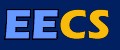# Geometric Continuity: A Parametrization Independent Measure of Continuity for Computer Aided Geometric Design

### http://www.eecs.berkeley.edu/Pubs/TechRpts/1986/CSD-86-255.pdf

Parametric spline curves and surfaces are typically constructed so that some number of derivatives match where the curve segments or surface patches abut. If derivatives up to order n are continuous, the segments or patches are said to meet with C^n, or nth order parametric continuity. It has been shown previously that parametric continuity is sufficient, but not necessary, for geometric smoothness.

The geometric measures of unit tangent and curvature vectors for curves (objects of parametric dimension one), and tangent plane and Dupin indicatrix for surfaces (objects of parametric dimension two), have been used to define first and second order geometric continuity. These measures are intrinsic in that they are independent of the parametrizations used to describe the curve or surface. In this work, the notion of geometric continuity as a parametrization independent measure is extended for arbitrary order n (G^n), and for objects of arbitrary parametric dimension p. Two equivalent characterizations of geometric continuity are developed: one based on the notion of reparametrization, and one based on the theory of differentiable manifolds.

From the basic definitions, a set of necessary and sufficient constraint equations is developed. The constraints (known as the Beta constraints) result from a direct application of the univariate chain rule for curves and the bivariate chain rule for surfaces. In the spline construction process the Beta constraints provide for the introduction of freely selectable quantities known as shape parameters. For polynomial splines, the use of the Beta constraints allows greater design flexibility through the shape parameters without raising the polynomial degree.

The approach taken is important for several reasons. First, it generalizes geometric continuity to arbitrary order for both curves and surfaces. Second, it shows the fundamental connection between geometric continuity of curves and that of surfaces. Third, due to the chain rule derivation, constraints of any order can be determined more easily than using derivations based exclusively on geometric measures. Finally, a firm connection is established between the theory of differentiable manifolds and the use of parametric splines in computer aided geometric design.

BibTeX citation:

```@phdthesis{DeRose:CSD-86-255,
Author = {DeRose, Anthony D.},
Title = {Geometric Continuity:  A Parametrization Independent Measure of Continuity for Computer Aided Geometric Design},
School = {EECS Department, University of California, Berkeley},
Year = {1985},
Month = {Aug},
URL = {http://www.eecs.berkeley.edu/Pubs/TechRpts/1985/6081.html},
Number = {UCB/CSD-86-255}
}
```

EndNote citation:

```%0 Thesis
%A DeRose, Anthony D.
%T Geometric Continuity:  A Parametrization Independent Measure of Continuity for Computer Aided Geometric Design
%I EECS Department, University of California, Berkeley
%D 1985
%@ UCB/CSD-86-255
%U http://www.eecs.berkeley.edu/Pubs/TechRpts/1985/6081.html
%F DeRose:CSD-86-255
```